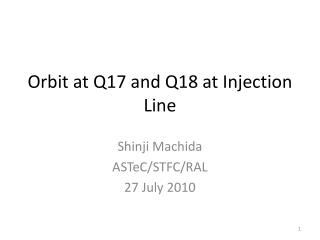DownloadDownload PresentationOrbit at Q17 and Q18 at Injection Line

# Orbit at Q17 and Q18 at Injection Line

Télécharger la présentation## Orbit at Q17 and Q18 at Injection Line

- - - - - - - - - - - - - - - - - - - - - - - - - - - E N D - - - - - - - - - - - - - - - - - - - - - - - - - - -
##### Presentation Transcript

1. Orbit at Q17 and Q18 at Injection Line Shinji Machida ASTeC/STFC/RAL 27 July 2010

2. Motivation • Mapping of septum, namely (x, x’) at exit as a function of (1) strength, (2) translation, (3) rotation, should be done with the identical (x, x’) at entrance. • There is no direct way to measure (x, x’) at entrance. • Instead of measuring (x, x’) at entrance, measure orbit at the last two quadrupoles just before septum.

3. Setup • To measure orbit at Q18 (focusing Q before BPM04), change Q18 excitation and measure position at BPM04. • To measure orbit at Q17 (defocusing Q before BPM04 and Q18), change Q17 excitation and measure position at BPM04. Q18 is off.  363 mm  177 mm  Q17 Q18 BPM04 septum

4. Formula • Deflection angle after going through a bending B • \theta = B L/(B \rho) = G x L/(B \rho) • Orbit displacement s at BPM after traveling distance of d • s = d \theta • Therefore • s = d G x L/(B \rho) = dx L c/(B \rho) I • where G [T/m] = c [T/m 1/A] I [A] and c = 1.5 • Measured data is orbit displacement “s” as a function of current “I”.

5. Fitting of data (1)orbit at Q18 • Slope = -0.057 mm/A for Horizontal (blue). • = +1.25 mm/A for vertical (red). • Orbit at Q18 is -0.16 mm in Hori and +3.4 mm in Vert.

6. Fitting of data (2)orbit at Q17 • Slope = -0.24 mm/A for Horizontal and (blue). • = -6.3 mm/A for vertical (red). • Orbit at Q17 is -0.22 mm in Hori and -5.7 mm in Vert.

7. Conclusion (1) • After the tuning of injection line on Wednesday 14 July 2010, a beam goes (with measurement accuracy) at the centre of Q17 and Q18 in horizontal, but not in vertical. • Because of different polarity of Q17 and Q18, vertical orbit displacement of -5.7 mm at Q17 and +3.4 mm at Q18 means on the same side.

8. Conclusion (2) • However, with nominal quadrupole setting, vertical at BPM04 is 0.27 to 0.30 mm. This is inconsistent with the orbits at Q17 and Q18. Axis of either quadrpoles or BPM04 may be off 2 - 3 mm.

9. Conclusion (3) • In principle, vertical orbit displacement does not affect septum mapping. However, we should try to make it zero for the next trial of septum mapping. • Alignment of quadrupoles and BPM may be necessary as well.